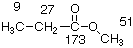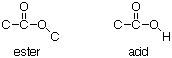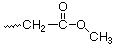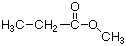Chapter 12

SPECTROSCOPIC STRUCTURE DETERMINATION:

A LIFELONG QUEST

INTRODUCTION

Why are there wiggles?

And why are there squiggles?

It’s all in the quantum mechanics...

Assume that you have a pure organic compound. How can you tell what it is? You can't tell just by looking at it - it usually looks like a blob of vegetable oil. Instead, you apply several spectroscopic techniques, and deduce from the data - the squiggles that the machine made on a piece of chart paper - what the structure is. This is immediately enjoyable, as within a week you will learn to decipher simple structures - and it can become a lifelong quest, figuring out more and more complicated structures.

THE QUEST, LEVEL ONE: FINDING THE PIECES

This I know, that whereas I was blind, now I see.

The pieces that make up an organic molecule are the ORGANIC FUNCTIONAL GROUPS and the HYDROCARBON FRAMEWORK. You will be able to find both the organic functional groups and the pieces of the hydrocarbon framework from the 13C NMR, the theory and practice of which are explained in detail in your lecture text.

As it comes from the spectrometer, a 13C NMR spectrum might look like this:Altogether, there are twelve lines in this spectrum. These can be divided into four sets: a single line at 173, four lines centered at 51, three lines centered at 27, and four lines centered at 9. The structure that gave rise to this spectrum is:There are four different carbons in this structure, labelled respectively 9, 27, 173 and 51. It can be seen that 173 gave a single line ( a singlet, s) in the 13C NMR spectrum, 51 gave four lines (quartet, q), 27 gave three lines (triplet, t), and 9 also gave four lines (q). If there had been a carbon atom with one H on it, it would have given two lines (doublet, d).

This spectrum introduces two new terms, CHEMICAL SHIFT and MULTIPLICITY. Chemical shift is the distance removed from zero at which the center of the pattern of lines appears (in this case 9, 27, 51 and 173). Multiplicity is the number of lines in each pattern. We will summarize 13C NMR spectra in the following way:

9, q

27, t

51, q

173, s

So...how do we use this information to find the ORGANIC FUNCTIONAL GROUPS in the molecule? Actually, there are two kinds of organic functional groups, those that have sp2 or sp-hybridized carbons, and those that have only sp3 hybridized carbons. We will call the former UNSATURATED FUNCTIONAL GROUPS, and the latter, SATURATED FUNCTIONAL GROUPS. These include:It is easy to tell if a molecule has any UNSATURATED FUNCTIONAL GROUPS, by considering the MOLECULAR FORMULA. In this example, the molecular formula is C4H8O2. A saturated hydrocarbon will have the formula CnH2n+2. If the ends of the hydrocarbon meet to form a ring, or if two H’s are removed to make an alkene, the formula becomes CnH2n. This count is not affected by oxygen. In our example, one pair of H's is missing, so there is one ring or double bond in the molecule - we can be sure that we have one unsaturated functional group. The IHD (Index of Hydrogen Deficiency) is the number of double bonds plus rings in the molecule. For C13H16O2, for instance, the IHD = 6. There are other considerations. While oxygen does not affect the IHD, nitrogen does. Because N is trivalent, each nitrogen brings with it an extra hydrogen. For instance, the IHD of C9H14N2O2 is four. The H14 includes two extra H’s, one for each of the N’s. Halogens (F, Cl, Br, I) in the formula count as H’s.

A double bond can be either a C=C bond or a C=O bond. There is O in the formula of our example, so there could be a C=O bond. From the examples above (there are more examples on pp. 212-213), we know that the C=O carbon of a ketone or aldehyde will come around 210, while the C=O carbon of an ester or acid will come around 170. Since we observe a singlet at 173, and we have two O's in the formula, we know that we have either an ester or an acid - but, which is it?A key difference between an ester and an acid is that with an ester, all of the H’s are attached to C. With an acid, one of the H’s is attached to an O. We can count in the 13C NMR spectrum how many H’s are attached to each C by the multiplicity: s = 0 H, d = 1H, t = 2 H, q = 3 H. In this example, if we add up all the H’s attached to C the total comes to 8. That is the same number of H’s as in the formula, so there are no H's attached to O. Therefore, the UNSATURATED FUNCTIONAL GROUP in this example must be an ester.

Next, we EXPLORE AROUND the unsaturated functional group. An ester has two carbons attached to it, one connected to the sp2-hybridized C, and one connected to the sp3-hybridized O. We would like to know how many H’s are attached to each of these two C’s. From the pictures on p. 213, we find that the C that is attached to the sp3-hybridized O comes around 50 or 60. That must be the signal in our data at 51. That signal is a quartet (q), so there must be three H’s attached to the C that is attached to the sp3-hybridized O. From the same pictures on p. 213, we find that the C connected to the sp2-hybridized C comes in the range 20-35. We can conclude that this must be the signal from our data at 27. That signal is a triplet (t), so there must be two H's attached to the C that is connected to the sp2-hybridized C of the carbonyl. Putting all of this together gives us the following partial structure.SATURATED FUNCTIONAL GROUPS: After you are finished with all of the unsaturated functional groups, are there heteroatoms (O, N, S) in the formula that you have not yet dealt with? If so, now would be the time to find the saturated functional groups. In this example, we had 2 O’s in the formula, and we have already accounted for both of them, so in this case there are no saturated functional groups.

HYDROCARBON FRAMEWORK: Which signals in the carbon spectrum have you not yet accounted for? In this case, all that is left is "9, q" - so there is one more -CH3 to attach to the structure.

PUTTING IT ALL TOGETHER: In this case, there is only one way to connect the pieces, so the structure must beTo solve a given structure, it is necessary to assemble a significant amount of data in an orderly way. This effort can often be confusing. To aid in this process, we will use a worksheet. A sample of the worksheet is reproduced on p. 184. Especially in the early going, you may find it useful to make several copies of this worksheet, and write directly on them in working the problems.

You should now ready to work through examples A-C in Chapter 12 of the lab text.